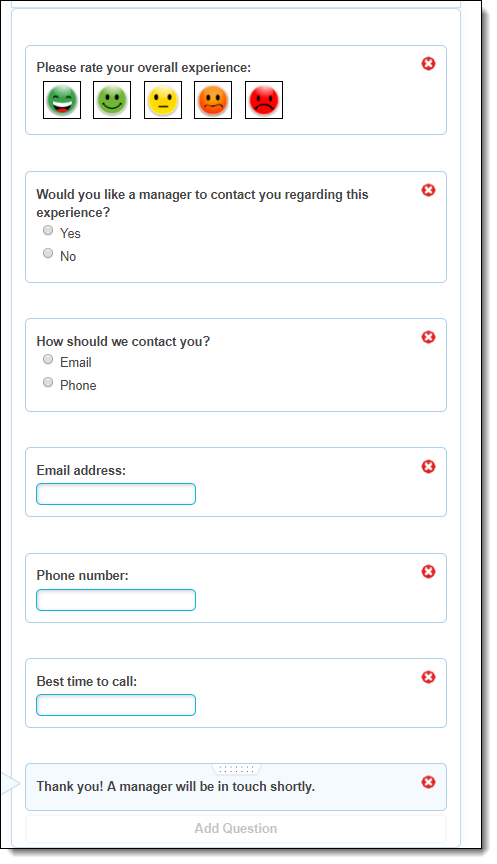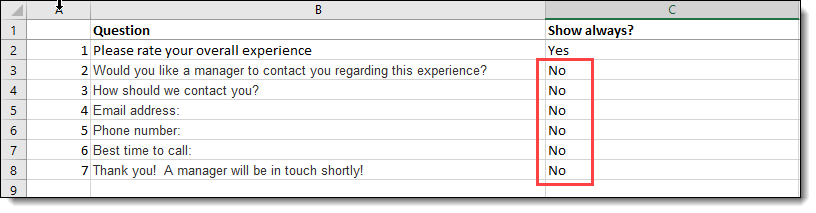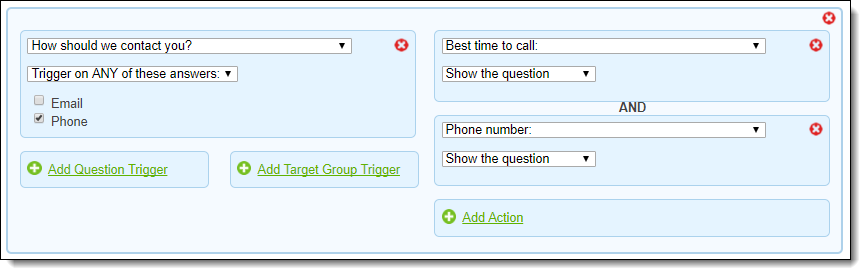Having problems with your Presto Logic?  You may be trying to do too much at once!  Read this article to learn how to handle complex situations in the simplest way possible.

Presto Logic is often used to control the flow of a survey through a series of decision points: if a question is answered a certain way, show another question, and if that question has a certain answer, show a third question.

Here's an example of a survey where we want the survey flow to "cascade" in this way:• Show "Would you like a manager to contact you?"
• If "Would you like a manager to contact you?" is answered Yes...
• Show "How should we contact you?"
• If "How should we contact you?" is answered Email...
• If "How should we contact you?" is answered Phone...
• Show "Phone number"
• Show "Best time to call"
• If EITHER "Email address" OR "Phone number" is answered, show "Thank you!  A manager will be in touch shortly."

If you're having problems with situations like this, it's probably because you're trying to do too much in a single Presto Logic rule.  Here's a method to break down your survey logically and identify all the conditions in which each question should be shown.

Step 1: List every question in the order in which they appear.  Using a spreadsheet helps, as does giving them numbers or some kind of shorthand (this doesn't need to be part of the survey text.

Step 2: Next to each question, list whether it always appears or not. Don't worry yet about specifying when it should appear -- just whether it always appears or not.Here's the important part: for every question that isn't always shown, you will need a Presto Logic rule.

Step 3: for each question that needs a rule, specify exactly when it should appear, with reference to preceding questions:This is the point where someone usually says, "But wait!  Shouldn't there be a rule that says, Show question 5 (Phone number) if question 1 is answered 'meh' to 'very sad' AND question 2 is answered 'Yes' AND question 3 is answered 'Phone'"?  The answer is no, because:

• You only want to show question 5 (Phone number) if question 3 is answered "Phone number", and
• Question 3 won't be shown (and thus can't be answered) unless question 2 is answered "Yes", and
• Question 2 won't be shown (and thus can't be answered) unless question 1 is answered "meh" to "very sad".

Get it?  The rules "cascade" -- so in each rule, you only want to do as much as you need to do to get to the next step.

Step 4: for every unique "Shown when?", create a Presto Logic rule

Construct each rule as follows::

• Under WHEN, add a question trigger that matches the question and response under "Shown when".  For example, from row 3 in the spreadsheet above, you'd add "shown when" question 1 (Please rate your overall experience) has any of the three answers shown below:• Under DO, add the question that you want to show (from column B) and the SHOW action:The resulting rule looks like this:Now repeat this for all the other rules in the spreadsheet.  Most of the rules look quite similar, but two are different.  One rule (if "How should we contact you?" is answered "Phone") shows and requires you to show TWO questions instead of one:...and, because we want to show question 7 when either question 4 or question 5, we'll make a rule with two question triggers instead of one:Note: because the triggering questions above are text questions, we can't create a rule based on a specific answer -- all we can do is specify that they have some answer.

That's it!  You have now created cascading Presto Logic rules!